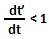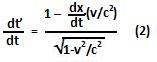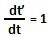## Friday, November 20, 2009

### Irodov Problem 1.355

First let us start by understanding the Lorentz transform. Lorentz transformation answers the following question. There are two observers O and O' with O moving at a speed v along the positive x=axis. If according to O a certain event occurs at (x,t), according to O' where (x') and when (t') does it occur?

In classical mechanics, the transformation would be x'=x-vt , t'=t. Since, the origin of O' has moved vt units forward along the x-axis with respect to that of O, all points would appear to have moved backwards along the x-axis by vt units, with respect to O'. Time elapsed of course is the same as perceived by O and O'.

In relativistic mechanics, time as perceived by the observers O and O' is not the same. The time would tick slower in O' compared to that of O as seen by O. In other words t' is less than t. The Lorentz transform tells us that,Let us understand (1). Suppose that the origins of the two frames (x,t) and (x',t') were initially aligned. After t seconds O' would have moved to x=vt as seen by O. Substituting this in (1) we have,This shows, that time t' as perceived by O' would be slower. This means that.

Differentiating (1) with respect to t we have,In order for the clocks to be in perpetual synchrony we must have that. From (2) we have,This means that if the observation point were displaced at this rate the clocks would always seem to be in Synchrony.